# Assignment 4, CSC 101

Parts 1 and 2 due February 11, 11:59pm
Parts 3, 4, and 5 due February 20, 11:59pm

This assignment pulls together the work of the previous assignments to create a functional (though somewhat hard-coded) ray casting program. In order to do so you will need to provide a few more data definitions and implement some functions (two required, but likely a few more as helper functions).

Unlike the previous assignments, your solution to this assignment will be built up in parts.

### Files

Create a hw4 directory in which to develop your solution. You will also need to copy your files from the previous assignment. Since the solution to this assignment is developed in parts, you may also choose to create subdirectories corresponding to each part.

You will develop the new parts of your program solution over three files. You must use the specified names for your files.

• `cast.py` - contains the function implementations
• `tests.py` - contains your test cases for individual functions (unit tests)
• `casting_test.py` - contains a main function and a scene cast test (system test)

Once you are ready to do so, and you may choose to do so often while incrementally developing your solution, run your test cases with the command python tests.py. To run your system test (to generate an image) use the command python casting_test.py > image.ppm.

### A Note on Color

The concept of a color appears in two different manifestations in this assignment. The majority of your code will work with a color as represented by red, green, and blue components that are `float` values. While, technically, such components should only range from 0.0 to 1.0, some of the arithmetic on colors (in the later parts) will allow for values greater than 1.0 (this is due to an "intensity" scaling factor).

The other representation of a color is strictly for the output format that is used in this assignment (the ppm P3 format discussed here). This representation requires integer values strictly in the range [0, 255] (for our purposes).

Converting between different representations is something that is often done in programming. For this assignment, all of your calculations are to be done in terms of `float` values. Then, just prior to printing, scale the color components so that they fall into the range [0, 255] (capping the values at 255), convert to an integer, and then print the components (you can write a separate utility function for converting).

## Part 1

For this part, you will implement a program that casts rays into a scene and prints an image. This image will be printed in version P3 of the ppm format. Details of this format are provided on a separate ppm P3 format page.

### Functions

For this first part you will implement simplified versions of the two primary functions for this assignment. In the later parts you will extend these functions.

#### Single Ray Cast

You must implement the primary casting function.

cast_ray(ray, sphere_list)

In `cast.py`, implement this function to "cast" the specified ray into the scene to gather all intersection points (and the corresponding spheres); this will use the appropriate function from the previous assignment. If an intersection is found, then this function returns `True`, otherwise the function returns `False`.

#### All Rays Cast

You must implement the following function to cast all of the rays required for the entire virtual "scene".

cast_all_rays(min_x, max_x, min_y, max_y, width, height, eye_point, sphere_list)

In `cast.py`, implement this function. This function controls the ray casting for the entire scene. The function will cast a ray for each pixel in the output image. These rays are cast from the eye point to points on a view rectangle as discussed below. Each ray cast will determine the color (black or white for Part 1) for the corresponding pixel.

The `min_x`, `max_x`, `min_y`, and `max_y` arguments specify the bounds of the view rectangle (with `z`-coordinate 0.0). The `width` and `height` specify the dimensions of the resulting image and, as such, the number of pixels. The pixels will be evenly distributed over the view rectangle: specifically, there will be `width` evenly distributed `x` coordinates in the interval [`min_x`, `max_x`) and `height` evenly distributed `y` coordinates in the interval [`max_y`, `min_y`) where the top-left corner of the view rectange is at point <`min_x`, `max_y`>. You will likely note that this skews the image slightly to the left and top of the view rectangle, but that is fine.

Cast a ray from the eye through each of these evenly distributed points beginning with the top-left corner (<`min_x`, `max_y`>) and varying the `x`-coordinate before varying the `y`-coordinate (i.e., compute every pixel in a row before moving to the next row). For each ray cast, print to the screen (in the format discussed above for P3) black (red=0.0, green=0.0, blue=0.0 in the internal color format; red=0, green=0, blue=0 in the file color format) if the cast results in an intersection and white (red=1.0, green=1.0, blue=1.0 in the internal color format; red=255, green=255, blue=255 in the file color format) if it does not.

For instance, if `min_x` is -4, `max_x` is 4, `min_y` is -2, `max_y` is 2, `width` is 4, and `height` is 2 then the points through which the ray will be cast are as follows (in this order): <-4, 2> <-2, 2> <0, 2> <2, 2> <-4, 0> <-2, 0> <0, 0> <2, 0>.

This process is illustrated by the image below (where the camera is at the eye position).### Test Cases

In `tests.py`, write test cases for `cast_ray` and any auxiliary (helper) functions that you have written. You need not provide test cases for `cast_all_rays` because this function prints to the console. This will be tested in a different manner as discussed below.

### Casting Test

In `casting_test.py`, use `cast_all_rays` to create an image using the following configuration. Be sure to print the P3 header to the screen before casting the rays. The width and height are as specified and the maximum color value is 255.

Eye at <0.0, 0.0, -14.0>.
A sphere at <1.0, 1.0, 0.0> with radius 2.0.
A sphere at <0.5, 1.5, -3.0> with radius 0.5.
A viewport with minimum x of -10, maximum x of 10, minimum y of -7.5, maximum y of 7.5, width of 512, and height of 384.

When you run your program, redirect the output to a file named `image.ppm`. This is done by typing the following at the command prompt. (The first command, `ulimit`, which only needs to be run once per terminal session, is used to limit the size of the files generated. This will prevent a run-away program from filling your disk quota.)

ulimit -f 12000
python casting_test.py > image.ppm

The image generated from this part should look like the following.

Though this is not the image we ultimately want, it is a useful image. The image indicates where intersections occurred, but it does not give much information beyond that. In fact, it is hard to tell that the scene contains two spheres. On to the next part.

Note: You can compare your generated image to the "expected" image using tools discussed at the bottom of this page.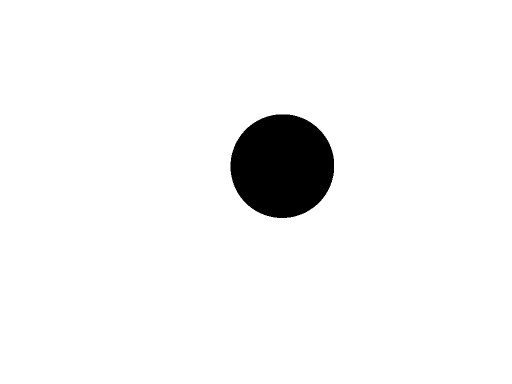A smaller image can be had by changing the viewport to the following (this is only for debugging purposes; the `casting_test.py` file that you submit must have the viewport set as originally specified).

Minimum x of 0.05859357, maximum x of 0.44921857, minimum y of 2.03125, maximum y of 2.421875, width of 20, and height of 20.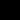## Part 2

To this point there is really nothing to make the spheres stand out. Let's add some color.

### Data Definitions

1. Modify `data.py` to add a new class to represent a `Color` in RGB (red, green, blue) format. The `Color.__init__` function should take arguments `r`, `g`, and `b` and initialize the corresponding attributes of the object. Be sure to define the `__eq__` function for this class.

2. Modify `Sphere.__init__` to take `color` (as its last argument) and to set the corresponding attribute of the object.

### Functions

1. In `cast.py`, update the `cast_ray` function to return the color of the sphere with the nearest intersection point (to the ray's origin), if there is an intersection, or a default color of white (1.0, 1.0, 1.0) if there is no intersection. Note: Do not make any assumptions about the order of the spheres in the array as relates to the nearness of the spheres.

### Test Cases

Update your test cases in `tests.py` to reflect the changes to these functions. Consider a test case for `cast_ray` that verifies it will find the nearest sphere regardless of the order of the spheres in the input array.

### Casting Test

Update `casting_test.py` so that the smallest sphere is red and the largest sphere is blue. The image generated from this part should look like the following.

It is now apparent that there are two spheres in the scene (perhaps more, if some are hidden behind the others, but we know there are only two in this scene). Unfortunately, these spheres still look like circles. On to the next part.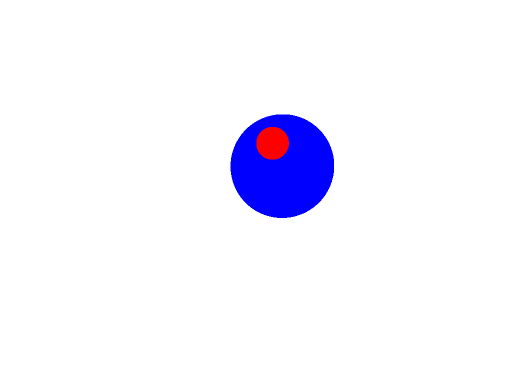And the smaller portion should look as follows.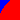## Part 3

In order to give the scene some depth, and in so doing make the spheres actually look like spheres instead of circles, we need to add some notion of light. This will be done over these last three parts. Each part will add one component of the light model.

In order to implement the light you will need to add a finish to the spheres in the scene. The idea being that the finish "describes" how the object interacts with light (e.g., some objects are shiny while others are dull).

### Data Definitions

1. Modify `data.py` to add a new class to represent a `Finish`. The `Finish.__init__` function should, for now, take a single argument `ambient` and initialize the corresponding attribute of the object. This attribute represents the percentage of ambient light reflected by the finish. Be sure to define the `__eq__` function for this class.

2. Modify `Sphere.__init__` to take `finish` (as its last argument) and to set the corresponding attribute of the object.

### Functions

1. In `cast.py`, update `cast_all_rays` so that it expects a `Color` as its last argument. This argument is the ambient light color.

2. In `cast.py`, update `cast_ray` so that it expects a `Color` as its last argument. This argument is the ambient light color.

3. Update `cast_ray` to modify the calculation of the color of the intersection point. If the ray does not intersect a sphere, then the returned color will remain the white default (1.0, 1.0, 1.0). If, however, the ray does intersect a sphere, then the returned color will now depend on the light model. At this point, the only contribution to the color will come from the reflected ambient light (think of the ambient light as a light that reaches everything).

Compute: Each component of the returned color will be computed by multiplying the corresponding component of the sphere's color by the ambient value of the sphere's finish and by the ambient light's color.

### Test Cases

Update your test cases in `tests.py` to test any newly created functions and to reflect the changes to existing functions.

### Casting Test

Update `casting_test.py` so that the scene's ambient light is white (1.0, 1.0, 1.0; though you may experiment with other light values, be sure to use this color for your submission). In addition, the large sphere should have a finish with ambient value `0.2` while the small sphere should have a finish with ambient value `0.4`. The image generated from this part should look like the following.

This just looks like a darker version of the previous image but that is fine. The fact is, all that we really did in this step was set up the framework for the next steps and soften the color based on the ambient scale in each sphere's finish (if you increase the ambient scale, you can get the same image as in the previous step, but leave the values as directed for submission).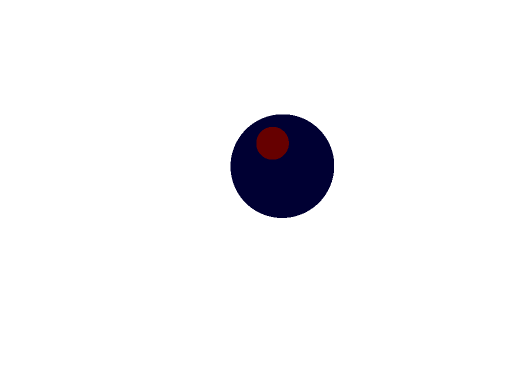And the smaller portion should look as follows.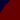## Part 4

For this step we will finally add some depth to the scene. This is accomplished by placing a point-light in the scene. Unlike the ambient lighting from the previous step, the point-light will emit light from a specified position.

### Data Definitions

1. In `data.py`, update `Finish.__init__` by adding `diffuse` as its last argument and by setting the corresponding attribute in the object. This attribute represents the percentage of diffuse light reflected by the finish. Be sure to update the `__eq__` function to reflect this change.

2. In `data.py`, add a new class to represent a `Light`. The `Light.__init__` function must take `pt` (a `Point` representing the position of the light) and `color` (a `Color` representing the color/intensity of the light) arguments and must initialize the corresponding attributes of the object. Be sure to define the `__eq__` function for this class.

### Functions

1. In `cast.py`, update `cast_all_rays` so that it expects a `Light` as its last argument.

2. In `cast.py`, `cast_ray` so that it expects a `Light` as its last argument.

3. Update `cast_ray` to modify calculation of the color of the intersection point. In addition to the ambient component of the color, a sphere intersection will now also compute a diffuse component that contributes (additively, i.e., the result of the following calculation is added to the result of the prior calculation) to the resulting color.

Consider the following options relating the intersection point on the sphere to the location of the point-light.

Note carefully that due to the imprecision of floating point values, using the computed intersection point in the following calculations can lead to unintended collisions with the sphere on which the point lies. To avoid these issues, these calculations will use a point just off of the sphere. This can be found by translating the intersection point along the sphere's normal (at the intersection point) by a small amount (use 0.01) (i.e., scale the normal by 0.01 to get a shorter vector and then translate the intersection point along this shorter vector). The following discussion refers to this new point as `pε`.

• Compute `pε` as just discussed.

• If the light is on the opposite side of the sphere from `pε`, then it does not contribute to the color of this point. This can be determined by computing the dot product of the sphere's normal at the intersection point with a normalized vector from `pε` to the light's position. If the light is in the general direction of the sphere's normal (i.e., on this side of the sphere), then the dot product will be positive. If the dot product is non-positive (i.e., 0 or negative), then we will consider the light to be on the opposite side of the sphere's surface and, thus, it will not hit this point (so the diffuse light contribution is 0).

1. Compute the sphere's normal at the intersection point. I will refer to this as `N`. (This was already computed in order to compute `pε` as described above).

2. Compute a normalized vector from `pε` to the light's position. I will refer to this as `Ldir`.

3. Compute the dot product of `N` and `Ldir` and use this value to determine if the light is "visible" from point `pε`.
• If another sphere is on the path from `pε` to the light's position, then the light is obscured and does not contribute to the color of this point. This can be determined by checking if a ray from `pε` in the direction of `Ldir` (computed above) collides with a sphere closer to `pε` than the light is.

• If the light is not obscured, then it will contribute to the point's color based on the diffuse value of the sphere's finish and the angle between the sphere's normal (at the intersection point) and the normalized vector pointing to the light.

More specifically, the light's diffuse contribution to the color of the point is (for each color component red, green, and blue) the product of a) the dot product between the sphere normal and the normalized light direction vector (i.e., `N ˙ Ldir`), b) the light's color component, c) the sphere's color component, and d) the diffuse scale of the sphere's finish.

### Test Cases

Update your test cases in `tests.py` to test any newly created functions and to reflect the changes to existing functions.

### Casting Test

Update `casting_test.py` so that the point-light is located at <-100.0, 100.0, -100.0> with color component (1.5, 1.5, 1.5). In addition, the finish for each sphere should have diffuse value `0.4`. The image generated from this part should look like the following.

You can now see how light provides a depth to the objects in the scene giving an illusion of a third-dimension. You can also see evidence of the shadow effect caused when a sphere partially obscures the light from hitting another sphere.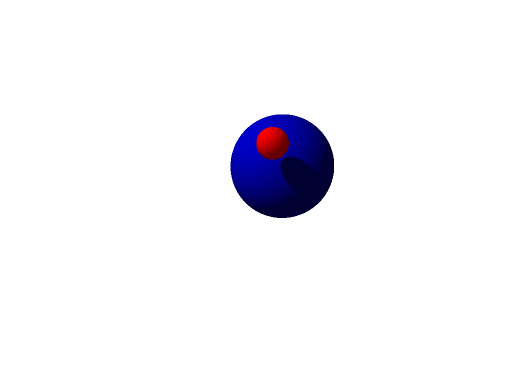And the smaller portion should look as follows.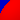## Part 5

This last step adds some additional character to the image through greater distinctions between different finishes by computing a specular component to the light model.

### Data Definitions

1. In `data.py`, update `Finish.__init__` to take `specular` and `roughness` as its last two arguments (in this order) and to set the corresponding attributes in the object. These attributes represent the percentage of specular light reflected by the finish and the modeled roughness of the finish (which affects the spread of the specular light across the object). Be sure to update the `__eq__` function to reflect this change.

### Functions

1. In `cast.py`, update `cast_ray` so that it expects a `Point` as its last argument. This is the position of the eye in the scene.

2. In `cast.py`, update `cast_ray` to modify the calculation of the color of the intersection point. In addition to the ambient and diffuse components of the color, a sphere intersection will now also compute a specular component that contributes (additively) to the resulting color.

The specular contribution is computed as follows.

1. Compute the specular intensity by computing the vector that represents the direction in which the light is reflected and determining the degree to which that light is aimed toward the eye. You can do this as follows.

1. Create a normalized vector from `pε` to the light's position (you already did this in the previous part). I will refer to this as the light direction, `Ldir`.

2. Compute the dot product of the light direction and the sphere's normal at the point of intersection (you already did this in the previous part). I will refer to this as the light normal dot, `LdotN`.

3. Compute the reflection vector as `Ldir - (2 * LdotN) * N`, where `N` is the sphere's normal at the point of intersection.

4. Compute a normalized vector from the eye position to `pε`. I will refer to this as the view direction, `Vdir`.

5. The specular intensity is the dot product of the reflection vector and `Vdir`.

2. If the specular intensity is positive, then the contribution to the point's color is the product of the light's color component, the specular value of the sphere's finish, and the specular intensity raised to a power of [1 divided by the roughness value of the sphere's finish]. If the specular intensity is not positive, then it does not contribute to the point's color.

### Test Cases

Update your test cases in `tests.py` to test any newly created functions and to reflect the changes to existing functions.

### Casting Test

Update `casting_test.py` so that the for each sphere has specular value `0.5` and roughness value `0.05`.

You can now see specular highlights from the light.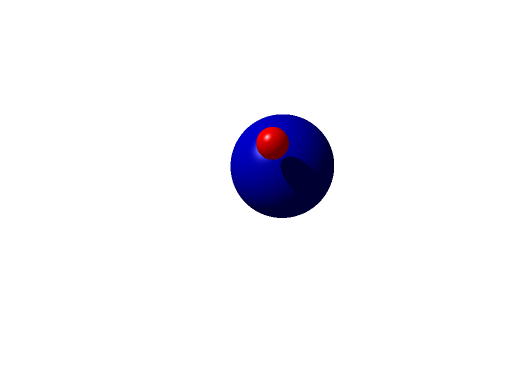And the smaller portion should look as follows.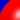## Handin

You must submit your solution on unix1.csc.calpoly.edu (or on unix2, unix3, or unix4) by 11:59pm on the due date.

The handin command will vary by section.

• Those in Aaron Keen's sections will submit to the akeen user.

To submit Parts 1 and 2, at the prompt, type handin akeen 101hw4_1_2 data.py vector_math.py collisions.py cast.py casting_test.py tests.py utility.py

To submit your completed assignment, at the prompt, type handin akeen 101hw4 data.py vector_math.py collisions.py cast.py casting_test.py tests.py utility.py

• Those in Julie Workman's sections will submit to the grader-ph user.

To submit Parts 1 and 2, at the prompt, type handin grader-ph 101hw4_1_2 data.py vector_math.py collisions.py cast.py casting_test.py tests.py utility.py

To submit your completed assignment, at the prompt, type handin grader-ph 101hw4 data.py vector_math.py collisions.py cast.py casting_test.py tests.py utility.py

• Those in Paul Hatalsky's sections will submit to the grader-ph user.

To submit Parts 1 and 2, at the prompt, type handin grader-ph 101hw4_1_2 data.py vector_math.py collisions.py cast.py casting_test.py tests.py utility.py

To submit your completed assignment, at the prompt, type handin grader-ph 101hw4 data.py vector_math.py collisions.py cast.py casting_test.py tests.py utility.py

• All of John Campbell's sections will use the same directory; submit to the jcampb25 user.

To submit Parts 1 and 2, at the prompt, type handin jcampb25 hw4_1_2 data.py vector_math.py collisions.py cast.py casting_test.py tests.py utility.py

To submit your completed assignment, at the prompt, type handin jcampb25 hw4 data.py vector_math.py collisions.py cast.py casting_test.py tests.py utility.py

Also, please handin any additional files, if any, that are required for your program to run.

Please note: the handin directory for parts 1 and 2 is different than the handin directory for the completed assignment.

Be sure to submit all files that are necessary to compile your program (including your files from the previous assignment(s)).

Note that you can resubmit your files as often as you'd like prior to the deadline. Each subsequent submission will replace files of the same name.

The grading breakdown for this assignment is as follows.

• Clean Execution: 5% — Program runs without crashing (and the submitted source demonstrates a legitimate attempt at a solution).

• Test Cases: 10% — Test cases are provided for each of the implemented functions. The number of test cases is appropriate for the complexity of the corresponding function (with a minimum of two test cases). The submitted test cases should be sufficient for the latest part completed (as submitted).

• Style: 5% — Consistent formatting, reasonable variable names, and each line of code below maximum length (i.e., less than 80 characters).

• Decomposition: 10% — Functional decomposition restricts each function to a single task. Duplicate functionality has been abstracted to a single function (i.e., duplicate code is removed).

• Functionality: 70% — Required functionality has been implemented.

### Sample Files and Comparing

Sample output images are given below (the earlier images shown in the browser are in a different format and are scaled down; do not compare against these). These are the images that should be generated by your system test for each part.

#### Image Diff Programs

You can compare your resulting image to those of the instructor solution with the following programs. Keep in mind, however, that there may be some legitimate minor differences due to the imprecision of floating point operations and the order in which your solution does computations compared to the order in the instructor solution.

There are two image diff programs available on the CSL machines. They are `~akeen/public/bin/ppmdiff` and `~akeen/public/bin/ppmdiff_analog`. Each of these programs takes two ppm P3 files as command-line arguments, reports the differences between the files to the console, and generates a difference image.

`ppmdiff` creates an image with a black pixel if the corresponding pixel position differs between the two input files. `ppmdiff_analog` creates an image with pixels colored by the degree to which the corresponding pixel position differs between the two input files. In short, the image from the first program highlights where the differences are while the image from the second program gives a sense of how "big" the differences are.